# Geometry Questions For CAT

Geometry questions for CAT are extremely important to prcatice as a lot of questions are included in the exam. As geometry is a very crucial topic for the CAT, candidates are expected to be well versed with all the related formulae and concepts to be able to solve the respective questions. So, here some practice CAT geometry questions are given for the candidates to help them to strengthen their CAT preparation.

## Important Geometry Questions for CAT:

1)In the regular hexagon given below, find the ratio of the area of the hexagon to the area of PQRV?

a) 5:1

b) 3:1

c) 12:5

d) 14:6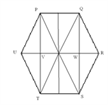2)What is the maximum number of times x circles of the same size can intersect each other?

a) 2x

b) x-1

c) x(x-1)

d) x(x+1)/2

3) Given that PQRS is a square. A line ST cuts the side QR at T and the diagonal PR at U such that RUS= 1000. Find angle UTR.

a) 600

b) 500

c) 550

d) cannot be determined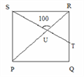4) If sin a= P and tan a= Q, find the value of 1/P2 -1/Q2 ?

a) 0

b) 1

c) 2

d) -1

5) A square is inscribed in a triangle with the two shorter sides p and q such that the square has a common right angle with the triangle. What is the perimeter of the square?

a) 4(1/p+1/q)

b) 4pq/(p+q)

c) (p2 q2)/(p+q)2

d) (1/p+1/q)2

6) Three balls of equal radius are placed such that they are touching each other. A fourth smaller ball is kept such that it touches the other three. Find the ratio of the radii of larger to smaller ball.

a) (2-v3)/v3

b) 3- 2×v3

c) 3.5

d) 4v5

7) What is the area of the largest triangle that can be fitted into a rectangle of length ‘l’ units and width ‘w’ units?

a) wl/3

b) wl/4

c) wl/2

d) none of these

8)A rectangle is 14 cm long and 10 cm wide. By what %age does the length change if we convert this into a square of the same area?

a) 10%

b) 20%

c) 15%

d) 12%

9)The sum of the areas of two circles which touch each other externally is 153p. If the sum of their radii is 15, find the ratio of the larger to the smaller radius.

a) 4

b) 2

c) 3

d) None of these

10)There is a circle of radius 10 units that circumscribes an equilateral triangle. A quadrilateral is drawn by joining midpoints of two adjacent sides of the triangle. Find the area of the quadrilateral

a) (225v3)/2

b) (225v3)/4

c) (225v3)/8

d) (225v3)/12

11) A rectangle is drawn so that none of its sides has a length greater than ‘x’. all lengths lesser than ‘x’ are equally likely. The chance that the rectangle has its diagonal greater than ‘x’ is (in %)?

a) 29.3%

b) 21.5%

c) 66.66%

d) 33.33%

12) A quadrilateral is obtained by joining the mid-points of a rectangle. Further, a rectangle is obtained by joining the mid points of quadrilateral obtained above. Again another quadrilateral is obtained by joining the mid points of the second rectangle.This process is repeated for an infinite number of times.Find the ratio of the sum of areas of all the rectangles to the sum of the areas of all the quadrilaterals.

a) 2 : 1

b) 3 : 1

c) 4 : 1

d) 2 : 3

13) If O is the center of a circle and the radius of the circle is 4 units, find the value of the length of AC.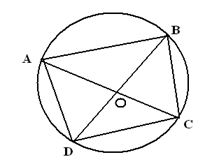a) 4( v3 + 1)

b) 9 v2

c) 10.5

d) noneof these

14)If ST is parallel to QR, and PS = SQ = 3 cm, PT = 4 cm and QR = 10 cm, what is the area of trapezoid STQR?

a) 12

b) 20

c) 30

d) 22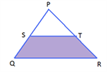15)If triangle PQS is an equilateral triangle and PQ = 6 and RT = 18,find the ratio of the shaded to the unshaded region.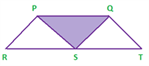a) 1:3

b) 1:3v3

c) 1:4

d) 2:5

16) Four concentric circles share the same center. The smallest circle has a radius of 1 inch. For n greater than 1, the area of the nth smallest circle in square inches, An, is given by An = An–1 + (2n – 1)p. What is the sum of the areas of the four circles, divided by the sum of their circumferences, in inches?

a) 1

b) 1½

c) 2

d) 2½

17) If one diagonal of the rhombus shown is 18 cm, then find the number of possibilities is there for the value of the side of the rhombus (only integral values)?

a) 0

b) 1

c) 2

d) 3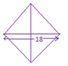18) If the right circular cone is cut into three solids of volumes V1,V2 and V3 by two cuts which are parallel to the base and trisects the altitude, then V1:V2:V3 is:

a) 1:2:3

b)1:4:6

c)1:6:9

d) None of these

19) In the figure find the area outside the trapezium, given square is of side 16 cm.

a)128 cm2

b)184 cm2

c)154 cm2

d)168 cm2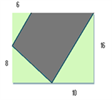20)A string of certain length n is made one perfect turn around a cube of side A, starting from corner X and ending at Y (as in the fig).

Find the length of the string.

a)v2A

b)v17 A

c)A

d)v17(A+1)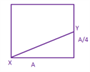21)A goat is tied to two poles P and Q with 15 m ropes. P and Q are 20 m apart. What is the area the goat is likely to graze?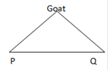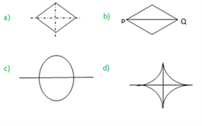22) AB is a line segment with P as the midpoint. Three semi circles are drawn with AP,PB and AB as diameters. Another circle is drawn so as to touch all three semi-circles. What’s the radius of this smaller circle?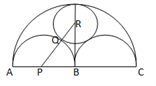a) 1/4 AB

b) 1/6 AB

c)1/7 AB

d)1/8 AB

Directions for questions 23-24 In the figure below, PR and QS intersect at right angles at point O.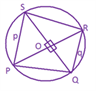23) Find the sum of areas of POQ and ORS

a) pq/2

b) (p2 q2)/2

c)(p2+q2)/2

d) (p2+q2)/8

24) The diameter of the circle on which the vertices of the quadrilateral lie is?

a) v(2p2 q)

b) (p2+q2)/v2

c)v(p2+q2 )

d) (p-q)/v2

25) Perpendiculars are dropped from the vertices of a triangle PQR on a line in the same plane as shown in the figure. The area of triangle PQR is?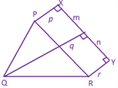a) [m(q-r)+n(q-p) ]/2

b) [m2 (q-r)+n2 (q-p) ]/2

c)[n2 (q-r)+m2 (q-p) ]/2

d) [m(q-r)+n(q+p) ]/2.

Problems Based On Cubes:

26) What is the maximum possible number of identical pieces a cube can be cut into by 9 cuts?

a) 9

b) 10

c) 13

d) 18

27) What is the least possible number of cuts required to cut a cube into 27 identical pieces?

a) 3

b) 2

c) 1

d) 8

28) A large cube is dipped into a tub filled with blue colour. When the cube is taken out it is observed that all its sides are painted. This large cube is now cut into 125 small but identical cubes. How many of the smaller cubes have none of its faces painted?

a) 27

b) 64

c) 8

d) none of these

Visit Geometry Solutions to check the detailed solution of the aforementioned CAT geometry questions.

Stay tuned with BYJU’S to get numerous practice question on important CAT exam topics. Also, get various preparation tips along with several CAT mock tests and sample papers to prepare better for the exam.